# Data Visualization with Python

visualization using python librariesPhoto by Isaac Smith on Unsplash

Hello everyone! In this article, I will guide you through simple data visualization techniques in Python using different libraries like matplotlib, seaborn . I assume you already know about why data visualization is so important and why we do. So without doing any theoretical explanations, I would like to make you aware of a few facts.

Before visualizing data you should always identify which type of data you are working on? Is it a numerical variable or categorical? Even in numerical is it continuous or discrete type of data? First, you can select a few visualization techniques for the type of data you are working on. You can easily do this after reading this article till the end.

The visualization process involves generally four steps:

1. Load and prepare the datasets: Normally you will pick a data set and visualize its observations. But the dataset must be cleaned first, filling of empty cells must be done, change categorical variables to numeric if necessary, and detecting outlier sometimes. If you clean the dataset before visualization the result will be more trustworthy.
2. Import the visualization libraries provided by python as per requirements. Most commonly used are Matplotlib and seaborn.
3. Plot the graph: After importing the libraries you will set many hyperparameters for size and display, and pass the datasets which will be visualized and then plot the diagram with proper syntax.
4. Display it on the screen. Finally, display the diagram.

Now let’s jump into the first visualization type.

# Line chart

A line chart is used to illustrate the relationship between two or more continuous variables. Rather than downloading a new dataset and cleaning lets just create a sample dataset using a python function. This will be a simple dataset with two columns. The first column will be date and second column will be Price, indicating the stock price on that date. This function will use radar python library to generate random data.

Here goes generateData() function:

`import datetimeimport mathimport pandas as pdimport randomimport radardef generateData(n): listdata = [] for _ in range(n):  date = radar.random_datetime(start='2019-08-1',  stop='2019-08-30').strftime("%Y-%m-%d")  price = random.uniform(900, 1000)  listdata.append([date, price]) df = pd.DataFrame(listdata, columns = ['Date', 'Price']) df['Date'] = pd.to_datetime(df['Date'], format='%Y-%m-%d') df = df.groupby(by='Date').mean() return df`

To run above generateData() function you must first install radar. In the above function, radar will create random 50 dates between dates specified with start and stop parameters. Then we grouped the dataframe ‘df’ with date. I assume you are aware of how groupby() function works. groupby() function is used for splitting, applying, and combining dataframe in pandas. For price values we used random.uniform() function which generates uniformly distributed values between 900 to 1000. So let’s see top 10 data of datatset we just created.

`df = generateData(50)df.head(10)`

The output of code above will be: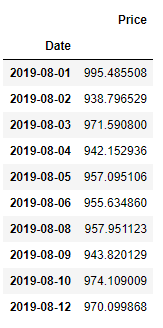average stock price on respective date

Now our dataset is ready so let’s import the matplotlib library and plot the line chart.

`import matplotlib.pyplot as pltplt.rcParams['figure.figsize'] = (14, 10)plt.plot(df)`

And the plotted graph looks something like this: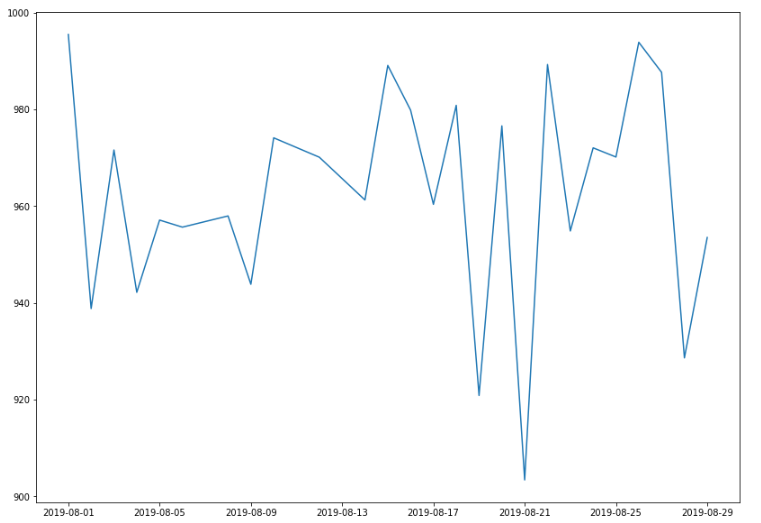Line plot for change in stock price

# Bar charts

Bar charts are one of the most common visualizations that almost everyone must have encountered since their school times. Bar charts are used for representing numbers in categorical variables like gender, marital status, months, individuals, blood type, etc. Bars can be drawn horizontally or vertically to represent categorical variables. Just like line chart let’s create a dataset for visualizing bar chart. As I already mention bar chart very well represents the categorical variable, let’s create a variable that will change over a period of months and visualize it on the basis of months.

Let’s assume a pharmacy in Norway keeps track of the amount of Zoloft sold every month. Zoloft is a medicine prescribed to patients suffering from depression. We can use the calendar Python library to keep track of the months of the year (1 to 12) corresponding to January to December.

Here we create variables with python libraries so let’s import necessary libraries first.

`import numpy as npimport calendarimport randomimport matplotlib.pyplot as pltmonths = list(range(1, 13))sold_quantity = [round(random.uniform(100, 200)) for x in range(1,13)]`

In this data, we just prepared both months and sold_quantity are list. Remember range stopping parameter is exclusive which means it doesn’t include the last item so it is (1–13) for 12 months. Now let’s Specify the layout of the figure and allocate space.

`figure, axis = plt.subplots()`

If no parameters are passed in matplotlib.pyplot it takes the default figure size, default axis. Here we set the values for figure size an axis of figure as subplots(). But on the x-axis, we would like to display the names of the months which will be done by the following code.

`plot = axis.bar(months, sold_quantity)`

After ploting we show the visualization with plt.show()

`plt.show()`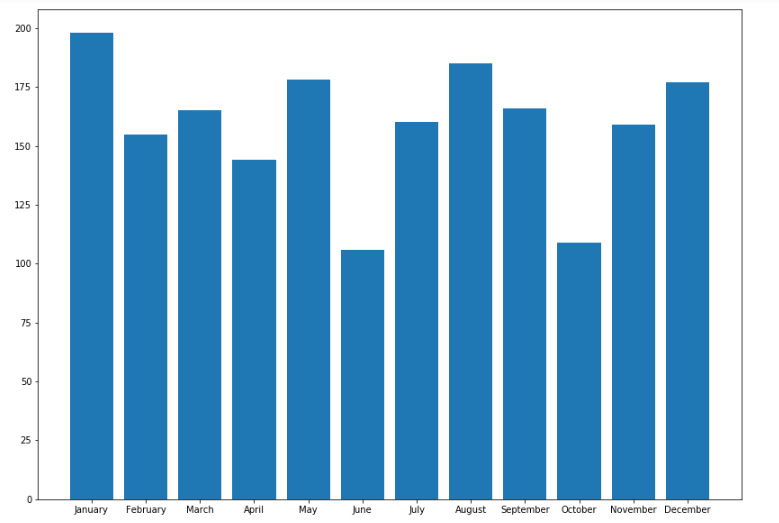Bar plot representing frequencies according to month

To plot this diagram you must run the above code in a single run. Let’s plot one more with the data value on the head of the bar. It will visually gives more meaning to show an actual number of sold items on the bar itself. And slightly changing the display of months’ names.

`plt.rcParams['figure.figsize'] = (14, 10)figure, axis = plt.subplots()plt.xticks(months, calendar.month_name[1:13], rotation=20)plot = axis.bar(months, sold_quantity)for rectangle in plot: height = rectangle.get_height() axis.text(rectangle.get_x() + rectangle.get_width() /2., 1.002 * height, '%d' % int(height), ha='center', va = 'bottom')plt.show()`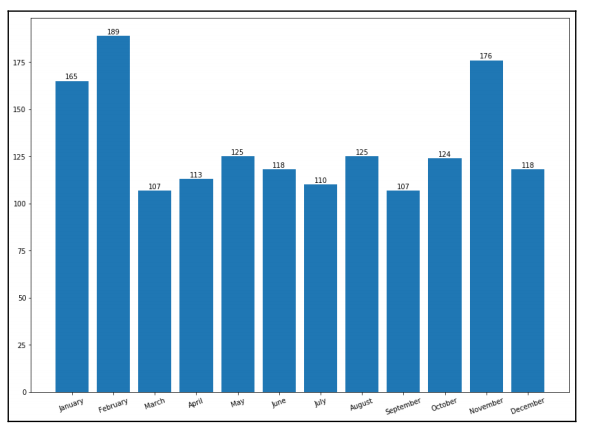Bar plot representing frequencies according to month

# Scatter plot

Scatter plot uses cartesian coordinates system to display values of typically two variables for a set of data. Scatter plots are generally constructed in the following two situations:

1. When one continuous variable is dependent on another variable:

Scatter plot is a very important technique while making sense of data. Any dataset that we want to analyze will have different fields/ columns of multiple observations/variables representing different facts. The columns of a dataset are, most probably related to one another because they are collected from the same event. One field of record may or may not affect the value of another field. To examine the type of relationships these columns have and to analyze the causes and effects between them, we have to work to find the dependencies that exist among variables. If such dependencies exist between tow columns than the scatter plot will clearly display it. So the scatter plot represents the relationship between an independent variable and a dependent variable.

Let’s plot a dataset into scatter plot which follows the concept of dependent and independent variables. Seaborn provides some mostly used datasets pre-loaded so you can directly load them when required. There is a dataset provided by seaborn called “tips” dataset. This is the record of bills of customer’s expenses and tips they gave. So first lets import necessary libraries for plotting scatter plot and set some default parameters of matplotlib.

`import seaborn as snsimport matplotlib.pyplot as pltplt.rcParams['figure.figsize'] = (8, 6)plt.rcParams['figure.dpi'] = 150sns.set()`

sns.set() will make some interesting style in diagram. Now load tips dataset.

`df = sns.load_dataset('tips')plt.scatter(x=df["total_bill"], y=df["tip"])plt.xlabel('Total bill')plt.ylabel('Tips given respectively')plt.show()`

Scatter plot requires variable for x-axis and y-axis compulsory. For a better understanding diagram we set the x-axis label and y-axis label as above. You can set other many different parameters for further more details in the diagram. Have a look at Matplotlib documentation for that. plt.show() will display the following visualization.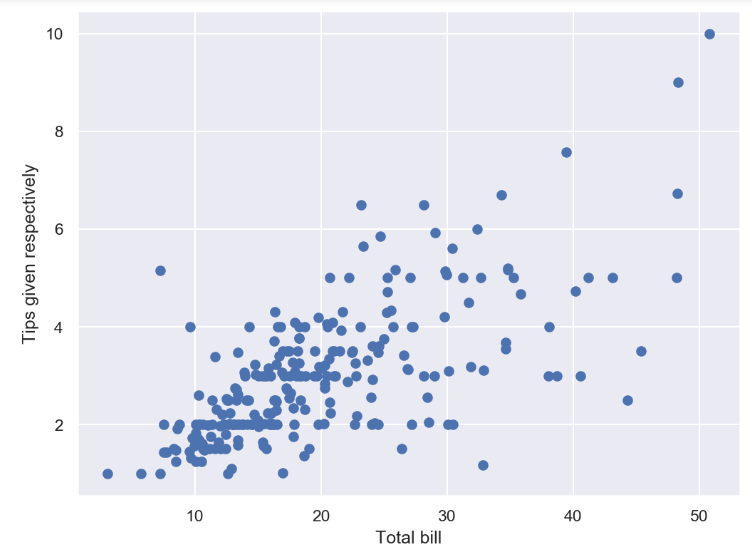scatter plot of total bill vs tips

As you can see, the more the bill, tips are higher. Here total bill amount is independent but the tips are slightly dependent on the amount of bill. If you wanna learn more on how to analyze this type of dependent and independent variable have a read of my building linear model article.

2. When both continuous variables are independent:
Let’s take an example of a scatter plot using the most popular dataset used in data science — the Iris dataset. This dataset is also available in seaborn pre-loaded. The dataset holds 50 examples each of three different species of Iris, named setosa, virginica, and versicolor. Each example has four different attributes: petal_length, petal_width, sepal_length, and sepal_width. So necessary libraries and default parameters are already set for scatterplot so let’s load iris dataset.

`df = sns.load_dataset('iris')df.head(10)`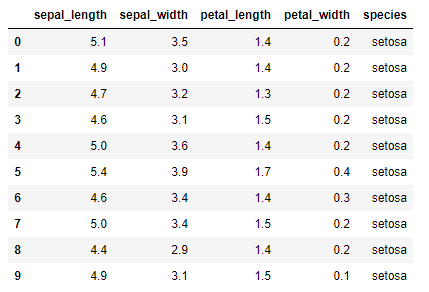look of first 10 records in dataset
`df['species'] = df['species'].map({'setosa': 0, "versicolor": 1,"virginica": 2})`

In this line of code above we modified categorical variable ‘species’ to dummy variables 0,1 and 2. As I already mentioned above about data preparation, this is one example. This df[‘species] column will come to use.

Next, create a regular scatter plot.

`plt.scatter(x=df[“sepal_length”], y=df[“sepal_width”], c = df.species)plt.xlabel(‘Septal Length’)plt.ylabel(‘Petal length’)plt.show()`

This code block will result the following scatter plot:

Here we also gave color parameter c, which will give color to our scatter points according to species. For a better understanding diagram we set the x-axis label and y-axis label as above.

# Histogram

Histogram plots are used to depict the distribution of any continuous variable. These types of plots are very popular in statistical analysis. Consider the following use cases. A survey created in vocational training sessions of developers had 100 participants. They had several years of Python programming experience ranging from 0 to 20. Let’s import the required libraries and create the dataset:

`import numpy as npimport matplotlib.pyplot as plt`

Now create a data set. Our data set will be an array of 100 variables assigned in ‘yearsOfExperience’.

`yearsOfExperience = np.array([10, 16, 14, 5, 10, 11, 16, 14, 3, 14, 13, 19,2, 5, 7, 3, 20,11, 11, 14, 2, 20, 15, 11, 1, 15, 15,15, 2, 9, 18, 1, 17, 18,13, 9, 20, 13, 17, 13, 15, 17, 10, 2, 11, 8, 5, 19, 2, 4, 9,17, 16, 13,18, 5, 7, 18, 15, 20, 2, 7, 0, 4, 14, 1, 14, 18,8, 11, 12, 2, 9, 7, 11, 2, 6, 15, 2, 14, 13, 4,6, 15, 3,6, 10, 2, 11, 0, 18, 0, 13, 16, 18, 5, 14, 7, 14, 18])`

Before ploting the histogram its better we already initialize the number of bins for this hstogram.

`nbins = 20plt.hist(yearsOfExperience, bins=nbins)plt.xlabel("Years of experience with Python Programming")plt.ylabel("Frequency")plt.title("Distribution of Python programming experience in the vocational training session")plt.show()`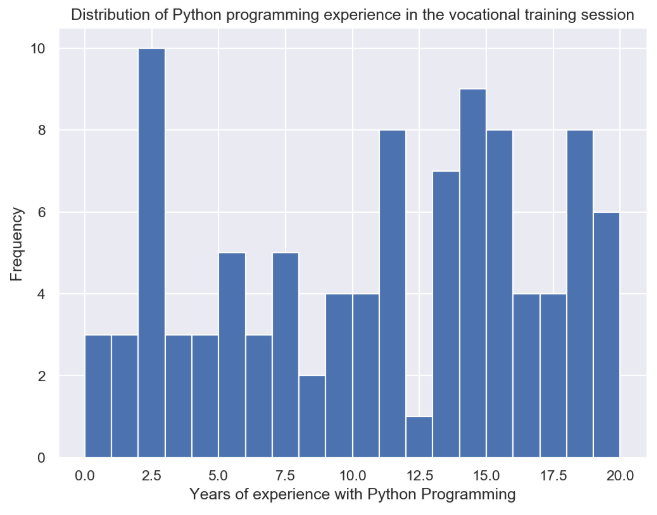Histogram for visualizing frequency of years of experience

Now, from the graph, we can say that the average experience of the participants is around 10 years.

These were the basics visualization techniques using matplotlib. Explaining every visualization method in a single article is not a great idea. you must first understand the purpose of visualization and then used the best visualization method for that purpose. Here is a table from Hands-on Explotary Data Analysis with python book.

This article is highly motivated by the book Hands on EDA with python . This book will teach you from introduction to EDA to implementing machine learning model with huge datasets .

: Hands-on Exploratory Data Analysis using Python, By Suresh Kumar Mukhiya, Usman Ahmed, 2020, PACKT Publication

--

--